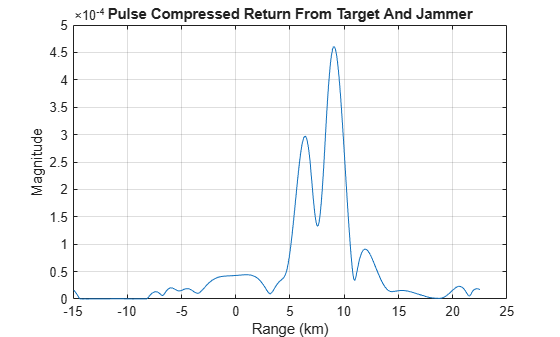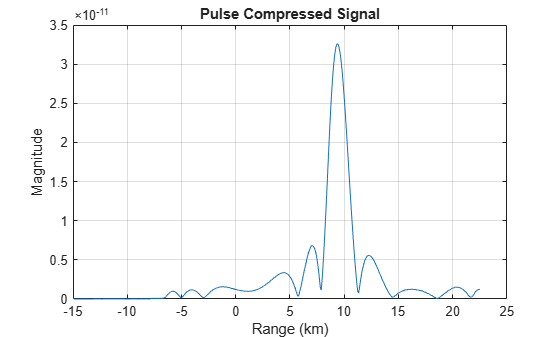# Frequency Agility in Radar, Communications, and EW Systems

This example shows how to model frequency agility in radar, communications and EW systems to counter the effects of interference.

### Introduction

Active electronically steered phased array systems can support multiple applications using the same array hardware. These applications may include radar, EW, and communications. However, the RF environments that these types of systems operate in are complex and sometimes hostile. For example, a repeater jammer can repeat the received radar signal and retransmit it to confuse the radar. In some literature, this is also called spoofing. Frequency agility can be an effective technique to counter the signals generated from interference sources and help support effective operations of these systems.

In this example, we first set up a scenario with a stationary monostatic radar and a moving aircraft target. The aircraft then generates a spoofing signal which confuses the radar. Once the radar detects the jamming source, frequency agility techniques can be employed which allows the radar to overcome the interference.

### System Simulation In an Interference Free Environment

Assume an X-band monostatic radar at the origin.

```fc = 10e9; % Define frequency of operation for X-band Radar fs = 2e6; % Define sample rate for the systems c = 3e8; lambda = c/fc; radar_pos = [0;0;0]; radar_vel = [0;0;0];```

The radar receiver, which can also function as an EW receiver, is a 64-element (8x8) URA with half wavelength spacing.

```% Array specification for the radar taper = taylorwin(8); taperURA = taper.*taper'; antenna = phased.URA('Element',phased.CosineAntennaElement,... 'Size',[8 8],'ElementSpacing',[lambda/2 lambda/2],... 'Taper',taperURA);```

The beam pattern of the array is shown in the following figure.

`pattern(antenna,fc,'Type','powerdb');`The radar transmits linear FM pulses. The transmitter and receiver specifications are:

```wav = phased.LinearFMWaveform('SampleRate',fs,... 'PulseWidth', 10e-5, 'SweepBandwidth', 1e5,'PRF',4000,... 'FrequencyOffsetSource','Input port'); tx = phased.Transmitter('Gain',20,'PeakPower',500); txArray = phased.WidebandRadiator('SampleRate', fs,... 'Sensor',antenna,'CarrierFrequency', fc); rxArray = phased.WidebandCollector('SampleRate', fs,... 'Sensor',antenna,'CarrierFrequency', fc); rxPreamp = phased.ReceiverPreamp('Gain',10,'NoiseFigure',5,... 'SampleRate', fs);```

The environment and target are described below. Wideband propagation channels are used to allow us to propagate waveforms with different carrier frequencies.

```target = phased.RadarTarget('MeanRCS',100,'OperatingFrequency',fc); target_pos = [8000;1000;1000]; target_vel = [100;0;0]; % Outgoing and incoming channel envout = phased.WidebandFreeSpace('TwoWayPropagation',false,... 'SampleRate', fs,'OperatingFrequency',fc,'PropagationSpeed',c); envin = phased.WidebandFreeSpace('TwoWayPropagation',false,... 'SampleRate', fs,'OperatingFrequency',fc,'PropagationSpeed',c);```

In this example, two one-way propagation channels are used because the jamming signal only propagates through the return channel.

The received echo can be simulated as

```rng(2017); [tgtRng, tgtAng] = rangeangle(target_pos, radar_pos); x = wav(0); % waveform xt = tx(x); % transmit xtarray = txArray(xt, tgtAng); % radiate yp = envout(xtarray,radar_pos,target_pos,radar_vel,target_vel); % propagate yr = target(yp); % reflect ye = envin(yr,target_pos,radar_pos,target_vel,radar_vel); % propagate yt = rxArray(ye,tgtAng); % collect yt = rxPreamp(yt); % receive```

We can perform a direction of arrival estimate using a 2D beam scan and used the estimated azimuth and elevation angles to direct the beamformer.

```estimator = phased.BeamscanEstimator2D('SensorArray',antenna,... 'DOAOutputPort',true,... 'OperatingFrequency', fc,... 'NumSignals',1,... 'AzimuthScanAngles',-40:40,... 'ElevationScanAngles',-60:60); [~,doa] = estimator(yt); beamformer = phased.SubbandPhaseShiftBeamformer('SensorArray',antenna,... 'OperatingFrequency',fc,'DirectionSource','Input port',... 'SampleRate',fs, 'WeightsOutputPort',true); [ybf,~] = beamformer(yt,doa);```

The beamformed signal can then be passed through matched filter and detector.

```mfcoeff1 = getMatchedFilter(wav); mf1 = phased.MatchedFilter('Coefficients',mfcoeff1); y1 = mf1(ybf); nSamples = wav.SampleRate/wav.PRF; t = ((0:nSamples-1)-(numel(mfcoeff1)-1))/fs; r = t*c/2; plot(r/1000,abs(y1),'-'); grid on; xlabel('Range (km)'); ylabel('Pulse Compressed Signal Magnitude');```The figure shows that the target produces a dominant peak in the received signal.

### Signal Analysis at the Target/Jammer

The radar works very well in the above example. However, in a complex environment, interferences can affect the radar performance. The interferences may be from other systems, such as wireless communication signals, or jamming signals. Modern radar systems must be able to operate in such environments.

A phased array radar can filter out interference using spatial processing. If the target and the jamming source are not closely located in angular space, beamforming may be an effective way to suppress the jammer. More details can be found in the Array Pattern Synthesis Part I: Nulling, Windowing, and Thinning example.

This example focuses on the situation where the target and the interference are closely located so that the spatial processing cannot be used to separate the two. Consider the case where the target aircraft can determine the characteristics of the signal transmitted from the radar and use that information to generate a pulse that will confuse the radar receiver. This is a common technique used in jamming or spoofing to draw the radar away from the true target.

The detected signal characteristics are displayed below

```pw = (pulsewidth(abs(yp), fs)); prf = round(1/pulseperiod(abs([yp;yp]), fs)); bw= obw(yp,fs,[],95); fprintf('Waveform Characteristics:\n');```
```Waveform Characteristics: ```
`fprintf('Pulse width:\t\t%f\n',pw);`
```Pulse width: 0.000100 ```
`fprintf('PRF:\t\t\t%f\n',prf);`
```PRF: 4000.000000 ```
`fprintf('Sweep bandwidth:\t%f\n',bw);`
```Sweep bandwidth: 112041.098255 ```

The jammer needs some time to do these analysis and prepare for the jamming signal so it is hard to create an effective spoofing signal right away, but generally within several pulses intervals the jamming signal is ready and the jammer can put it within arbitrary position in a pulse to make the spoofing target look closer or father compared to the true target. It is also worth noting that with the latest hardware, the time needed to estimate the characteristics of the signal decreases dramatically.

Assume the jammer wants to put the signal at about 5.5 km out, the jammer could transmit the jamming signal at the right moment to introduce the corresponding delay. In addition, because this is a one way propagation from the jammer to the radar, the required power is much smaller. This is indeed what makes jamming very effective as it does not require much power to blind the radar.

```jwav = phased.LinearFMWaveform('SampleRate',fs,... 'PulseWidth',pw,'SweepBandwidth',bw,'PRF',prf); xj = jwav(); Npad = ceil(5500/(c/fs)); xj = circshift(xj,Npad); % pad zero to introduce corresponding delay txjam = phased.Transmitter('Gain',0,'PeakPower',5); xj = txjam(xj); ye = envin(yr+xj,target_pos,radar_pos,target_vel,radar_vel); yt = rxArray(ye,tgtAng); yt = rxPreamp(yt); ybfj = beamformer(yt,doa); y1j = mf1(ybfj); % Jammer plus target return plot(r/1000,abs(y1j)); grid on; xlabel('Range (km)'); ylabel('Magnitude'); title('Pulse Compressed Return From Target And Jammer');```The received signal now contains both the desired target return and the jamming signal. In addition, the jamming signal appears to be closer. Therefore, the radar is more likely to lock on to the closest target thinking that one is the most prominent threat and spend less resource on the true target.

### Frequency Agility to Counter Interference

In the following situation, assume that the transmitted signal hops 500 kHz from the original carrier frequency of 10 GHz. Therefore, the new waveform signal becomes

```deltaf = fs/4; xh = wav(deltaf); % hopped signal ```

The figure below shows the spectrogram of both the original signal and the hopped signal. Note that the hopped signal is a shift in the frequency domain compared to the original signal.

`pspectrum(x+xh,fs,'spectrogram')`Using similar approach outlined in earlier sections, the radar echo can be simulated using the new waveform. Note that since jammer is not aware of this hop, the jamming signal is still the same.

```xth = tx(xh); xtharray = txArray(xth, tgtAng); yph = envout(xtharray,radar_pos,target_pos,radar_vel,target_vel); yrh = target(yph); yeh = envin(yrh+xj,target_pos,radar_pos,target_vel,radar_vel); yth = rxArray(yeh,tgtAng); yth = rxPreamp(yth); ybfh = beamformer(yth,doa);```

Because the hopping schedule is known to the radar, the signal processing algorithm could use that information to extract only the frequency band that around the current carrier frequency. This helps reject the interference at other bands and also improves the SNR since the noise from other bands are suppressed. In addition, when the waveform hops, the matched filter needs to be updated accordingly.

Let us now apply the corresponding bandpass filters and matched filters to the received signal.

First, create a bandpass filter using the signal's bandwidth.

`buttercoef = butter(9,bw/fs);`

Then, we can modulate the resulting bandpass filter with a carrier to obtain the bandpass filter around that carrier frequency.

`bf2 = buttercoef.*exp(1i*2*pi*deltaf*(0:numel(buttercoef)-1)/fs);`

Similarly, the matched filter coefficient needs to be modulated too.

```mfcoeff2 = getMatchedFilter(wav,'FrequencyOffset',deltaf); mf2 = phased.MatchedFilter('Coefficients',mfcoeff2); % extract bands and apply matched filters yb2 = mf2(filter(bf2(:),1,ybfh)); % plot the matched filtered signal plot(r/1000,abs(yb2)); grid on; xlabel('Range (km)'); ylabel('Magnitude'); title('Pulse Compressed Signal');```The figure shows that with the adoption of frequency hopping, the target echo and the jamming signal can be separated. Since the jammer is still in the original band, only the true target echo appears in the new frequency band where the waveform currently occupies, thus suppressing the impact of the jammer.

### Summary

This example shows that adopting frequency agility can help counter the jamming effect in a complex RF environment. The example simulates a system with frequency hopping waveform and verifies that this technique helps the radar system to identify the true target echo without being confused by the jamming signal.